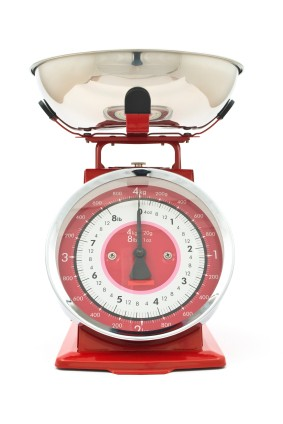# Measurement Abbreviations

Measurement abbreviations are not hard to master. They are the same for singular and plural forms of a word, which simplifies things significantly. For example, one kilometer is abbreviated 1 km, and two kilometers is 2 km. Let's take a look at the most often used measurement abbreviations and get a little insight into how these abbreviations are developed.

## Measurement Abbreviations

Here's a handy guide to more than 25 measurement abbreviations arranged by what they measure.

### Height/Length/Distance/Range/Depth

• ft - foot/feet. A foot is twelve inches.

• in - inch/inches. An inch is 1/12 of a foot.

• mm - millimeter/millimeters. "Milli" comes from the Latin word for one thousand; but, in measurements, it usually indicates one thousandth, not one thousand. A millimeter is one thousandth of a meter.

• cm - centimeter/centimeters. "Centi" means one hundred, but just like "milli" in measurements, it means one hundredth, not one hundred. A centimeter is one hundredth of a meter.

• m - meter/meters. A meter is equal to 39.37 inches, or slightly more than three feet.

• km - kilometer/kilometers. "Kilo" comes from the Greek word for one thousand. A kilometer is one thousand meters.

• yd - yard/yards. A yard is equal to three feet, or not quite a meter.

• mi - mile/miles. A mile is 5280 feet, 1760 yards, or approximately 1.6 kilometers.

### Weight

• lb - pound/pounds. "lb" comes from the Latin word for pound, "libra."

• oz - ounce/ounces. There are 16 ounces in a pound.

• mg - milligram/milligrams. A milligram is one thousandth of a gram.

• g - gram/grams. A gram is one thousandth of a kilogram.

• kg - kilogram/kilograms. A kilogram is equal to approximately 2.2 pounds.

### Volume/Capacity

• tsp or t - teaspoon/teaspoons. A teaspoon is equal to about 1/6 of a fluid ounce or almost five milliliters.

• tbs, tbsp or T - tablespoon/tablespoons. A tablespoon is about half a fluid ounce, three teaspoons or fifteen milliliters.

• c - cup/cups. A cup is half a pint or eight ounces.

• qt - quart/quarts. A quart is a quarter of a gallon, two pints or about a liter (more or less, depending on whether it's a US liter or a British liter).

• ml - milliliter/milliliters. A milliliter is one thousandth of a liter.

• l - liter/liters. A liter is a measure of capacity equal to 1000 cubic centimeters. If a container that size were filled with liquid, it would equal about 1.75 pints.

• pt - pint/pints. A pint is half of a quart.

• gal - gallon/gallons. A gallon is four quarts, or not quite four liters.

### Temperature

• F - degrees Fahrenheit

• cal - calorie (small calorie)

• Cal - kilocalorie (large calorie)

### Speed

• mph - miles per hour

• kph - kilometers per hour

• kn - knots. Nautical miles per hour. A nautical mile is about 2025 yards.

## Formation of Measurement Abbreviations

Measurement abbreviations are formed, for the most part, in one of three ways.

1. An abbreviation might be formed using the first and last letter of the complete word. "Yard," for example, is abbreviated "yd.," and "quart" is abbreviated "qt."

2. Some measurement abbreviations are formed by using the first letter or two (or sometimes three) of the word. Some examples of this are mi. (mile), in. (inch), cal. (calorie), and all of the basic units of measurement in the metric system (meter, gram and liter).

3. The third way measurement abbreviations are often formed is by using the first letter of each word (mph/miles per hour) or major part of the word. When a prefix is added to a basic metric unit, the word is abbreviated by using the first letter of the prefix and the first letter of the basic unit. "Centimeter" is centi + meter, so the abbreviation is "cm."

Once you have all these down, you'll be able to take shorthand notes for chefs, cartographers and apothecaries all the world over!Measurement Abbreviations